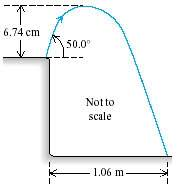# Question involving projectiles in motion.

## Homework StatementNow I'm pretty much an idiot, but the question to this is "Use information from the figure to find the initial speed of the grasshopper."

Now I know that I need time, but this is where I'm having a problem. How would I calculate time without velocity. If I had some equations I would be able to do it with no problem, but I'm pretty much making this harder than it should be... If anyone could set me up with some equations I could possibly try it out and run it by you guys!

:(

## The Attempt at a Solution

:(

cepheid
Staff Emeritus
Gold Member
Hi Jaime, welcome to PF!

The equations are just those of basic kinematics of projectile motion, but rather than focusing on that, let me walk you through it conceptually. You really do have all of the information you need:

- As soon as the grasshopper leaves the ground, it is in free fall (i.e. it is a projectile -- an object that moves solely under the influence of gravity)

- because of this first point, you know the grasshopper's acceleration is always g, downwards.

- Given the acceleration in the previous point, and the maximum height reached, you can figure out using basic kinematics what the initial vertical component of the velocity must have been at launch in order for the grasshopper to have reached that max height before decelerating to zero and starting to fall back down. You can also figure out how much time it takes for the grasshopper to reach this height

- Given the max height from which the grasshopper falls, you can figure out how much time it will take for for it to fall back down to the ground.

- Given the total 'air' time from the two previous points, you can figure out what the horizontal component of the velocity must have been in order for the grashopper to have travelled 1.06 m horizontally during that time. Recall that the horizontal component of the velocity is unaffected by gravity (the acceleration is entirely vertical, and the two components of the velocity are therefore independent of each other).

- Note, also, that the launch angle of 50 degrees tells you what the relationship between horizontal and vertical velocity is, meaning that you could have calculated the horizontal launch velocity as soon as you found out the vertical one, without going through those intermediate steps. It's nice that you have that additional info.

Thanks so much! I'm going through my equations, you broke it down so nicely!